Open In App

# Time Series Analysis using Facebook Prophet

Time series analysis is one of the important methodologies which helps us to understand the hidden patterns in a dataset that is too related to the time at which it is being recorded. In this article, we will first understand what is time series analysis and a method to do the same.

## What is Time Series Forecasting?

As we know time series data is data in which there is a dependency on the time with the data which is being observed. Predicting the future values of the data by analyzing the previous trends and the patterns hidden in the data is known as time series forecasting.

With time multiple time series analyses and forecasting techniques that have evolved in the market like ARIMA or SARIMA, one can also use deep learning-based methods like LSTM and RNN which are specially designed for performing forecasting and analysis of sequential data.

Prophet is an open-source tool from Facebook used for forecasting time series data which helps businesses understand and possibly predict the market. It is based on a decomposable additive model where non-linear trends fit with seasonality, it also takes into account the effects of holidays. Before we head right into coding, let’s learn certain terms that are required to understand this.

• Trend: The trend shows the tendency of the data to increase or decrease over a long period of time and it filters out the seasonal variations.
• Seasonality: Seasonality is the variations that occur over a short period of time and is not prominent enough to be called a “trend”.

## Understanding the Prophet Model

The general idea of the model is similar to a generalized additive model. The “Prophet Equation” fits, as mentioned above, trends, seasonality, and holidays. This is given by,

y(t) = g(t) + s(t) + h(t) + e(t)

where,

• g(t) refers to trend (changes over a long period of time)
• s(t) refers to seasonality (periodic or short term changes)
• h(t) refers to effects of holidays to the forecast
• e(t) refers to the unconditional changes that is specific to a business or a person or a circumstance. It is also called the error term.
• y(t) is the forecast.

### Why do we need a tool like Prophet to help us with forecasting?

We need it because, although the basic decomposable additive model looks simple, the calculation of the terms within is hugely mathematical. If you do not know what you are doing, it may lead to making wrong forecasts, which might have severe repercussions in the real world. So to automate this process, we are going to use Prophet. However, to understand the math behind this process and how Prophet actually works, let’s see how it forecasts the data.

Prophet provides us with two models(however, newer models can be written or extended according to specific requirements).

1. Logistic Growth Model
2. Piece-Wise Linear Model

By default, Prophet uses a piece-wise linear model, but it can be changed by specifying the model. Choosing a model is delicate as it is dependent on a variety of factors such as company size, growth rate, business model, etc., If the data to be forecasted, has saturating and non-linear data(grows non-linearly and after reaching the saturation point, shows little to no growth or shrink and only exhibits some seasonal changes), then logistic growth model is the best option. Nevertheless, if the data shows linear properties and had growth or shrink trends in the past then, the piece-wise linear model is a better choice. The logistic growth model is fit using the following statistical equation,

(1)where,

• C is the carrying capacity
• k is the growth rate
• m is an offset parameter

The piece-wise linear model is fit using the following statistical equations,

(2)where c is the trend change point(it defines the change in the trend). ? is the trend parameter and can be tuned as per requirement for forecasting.

Now let’s use this knowledge with a real example. Consider the air passengers data set(please open the link below and save the .csv file) https://raw.githubusercontent.com/rahulhegde99/Time-Series-Analysis-and-Forecasting-of-Air-Passengers/master/airpassengers.csv  The above data-set contains the number of air passengers in the USA from January 1949 to December 1960. The frequency of the data is 1 month. Now let’s try and build a model that is going to forecast the number of passengers for the next five years using time series analysis.

### Installing Prophet and Other Dependencies

Install pandas for data manipulation and for the dataframe data structure.

!pip install pandas

Install Prophet for time series analysis and forecasting.

!pip install fbprophet

Note: If you don’t want to install the modules locally, use Jupyter Notebooks.

## Python3

 import pandas as pdfrom fbprophet import Prophetfrom fbprophet.plot import add_changepoints_to_plot

Now let’s load the csv file in the panda’s data frame.

## Python3

 url = ("https://raw.githubusercontent.com/rahulhegde99"      "/Time-Series-Analysis-and-Forecasting-of-Air-Passengers"        "/master/airpassengers.csv")data = pd.read_csv(url)data.head()

Output: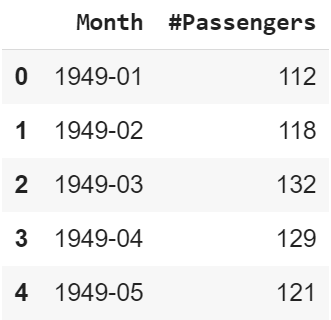First five rows of the dataset

Facebook Prophet predicts data only when it is in a certain format. The dataframe with the data should have a column saved as ds for time series data and y for the data to be forecasted. Here, the time series is the column Month and the data to be forecasted is the column #Passengers. So let’s make a new dataframe with new column names and the same data. Also, ds should be in a DateTime format.

## Python3

 df = pd.DataFrame()df['ds'] = pd.to_datetime(data['Month'])df['y'] = data['#Passengers']df.head()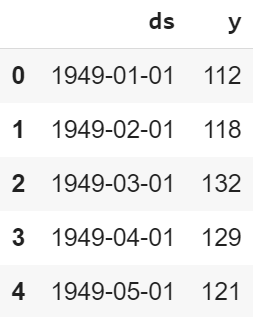First five rows of the dataset

### Initializing a Prophet Model

By using the Prophet() command we can initialize an instance of the fbprophet model for the training on our dataset and then help us to perform time series forecasting.

## Python3

 m = Prophet()m.fit(df)

Output:

<fbprophet.forecaster.Prophet at 0x7fd6e50b97c0>
99       401.676    0.00247936       124.055           1           1      130
Iter      log prob        ||dx||      ||grad||       alpha      alpha0  # evals  Notes
149       401.793   5.51406e-06       75.3197   8.648e-08       0.001      238  LS failed,
199       401.842   1.49744e-05       84.6086      0.3727           1      300  Hessian reset
Iter      log prob        ||dx||      ||grad||       alpha      alpha0  # evals  Notes
299       401.855   1.97478e-05        85.557           1           1      416
Iter      log prob        ||dx||      ||grad||       alpha      alpha0  # evals  Notes
399       401.857   2.66739e-05       75.1658           1           1      540
Iter      log prob        ||dx||      ||grad||       alpha      alpha0  # evals  Notes
499       401.978   3.52064e-07       67.2392           1           1      667
Iter      log prob        ||dx||      ||grad||       alpha      alpha0  # evals  Notes
508       401.978   2.80324e-06       94.3982   3.938e-08       0.001      717  LS failed,
533       401.978   6.17194e-09       67.4932     0.03341           1      753  Hessian reset
Optimization terminated normally:
Convergence detected: absolute parameter change was below tolerance

We want our model to predict the next 5 years, that is, till 1965. The frequency of our data is 1 month and thus for 5 years, it is 12 * 5 = 60 months. So we need to add 60 to more rows of monthly data to a dataframe.

## Python3

 future = m.make_future_dataframe(periods=12 * 5,                                 freq='M')

Now in the future dataframe we have just ds values and we should predict the y values.

## Python3

 forecast = m.predict(future)forecast[['ds', 'yhat', 'yhat_lower',          'yhat_upper', 'trend',          'trend_lower', 'trend_upper']].tail()

Output: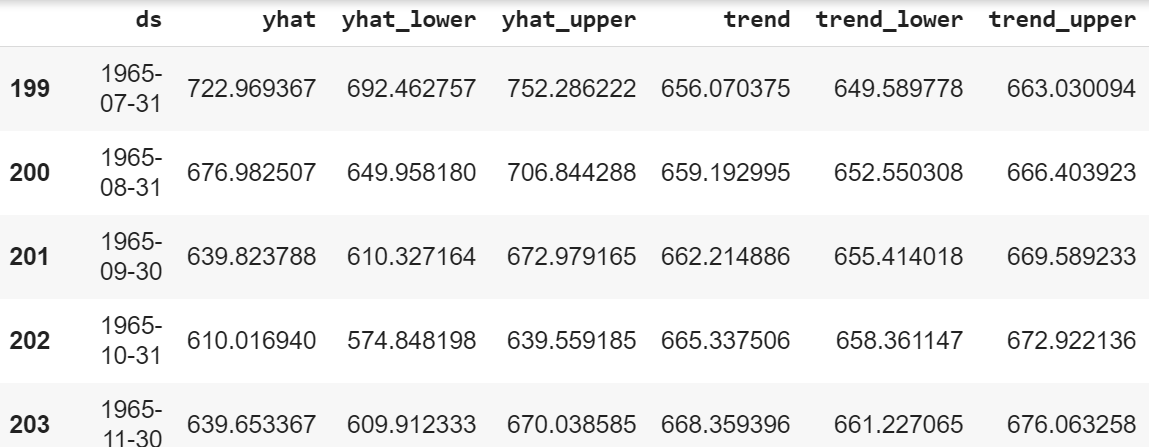Forecast data in the dataframe

### Plotting the Forecast Data

Table ds, as we know, is the time series data. yhat is the prediction, yhat_lower, and yhat_upper are the uncertainty levels(it basically means the prediction and actual values can vary within the bounds of the uncertainty levels). Next up we have a trend that shows the long-term growth, shrink, or stagnancy of the data, trend_lower, and trend_upper is the uncertainty levels.

## Python3

 fig1 = m.plot(forecast)

Output: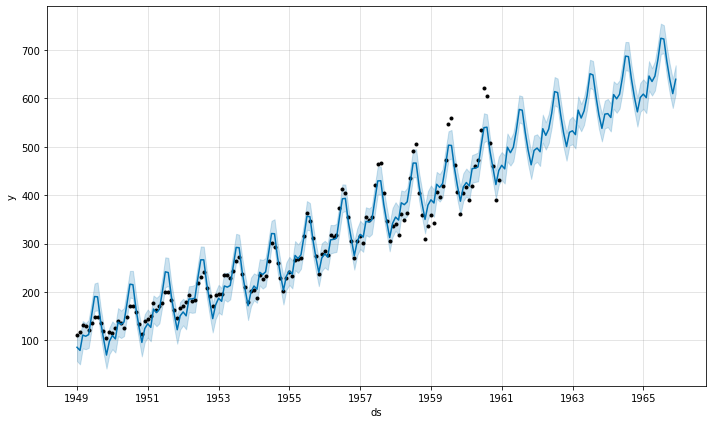Prediction of Air Passengers in the USA

The below image shows the basic prediction. The light blue is the uncertainty level(yhat_upper and yhat_lower), the dark blue is the prediction(yhat) and the black dots are the original data. We can see that the predicted data is very close to the actual data. In the last five years, there is no “actual” data, but looking at the performance of our model in years where data is available it is safe to say that the predictions are close to accurate.

## Python3

 fig2 = m.plot_components(forecast)

Output: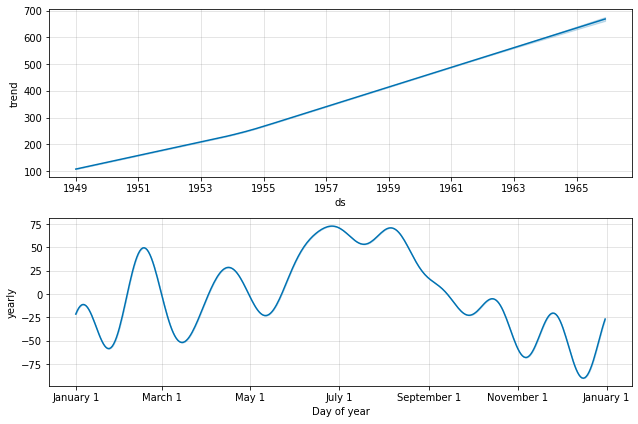Trends and Seasonality in Time Series Data

The below images show the trends and seasonality(in a year) of the time series data. We can see there is an increasing trend, meaning the number of air passengers has increased over time. If we look at the seasonality graph, we can see that June and July is the time with the most passengers in a given year.

## Python3

 fig = m.plot(forecast)a = add_changepoints_to_plot(fig.gca(),                             m, forecast)

Output: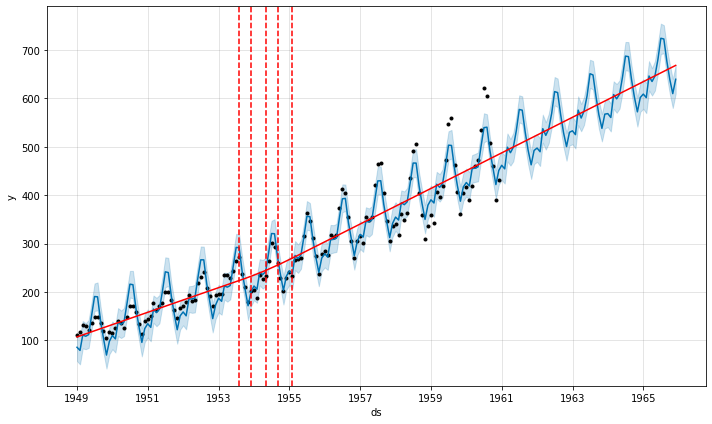Air Passengers with trend changepoint

Add changepoints to indicate the time in rapid trend growths. The dotted red lines show the time when there was a rapid change in the trend of the passengers. Thus, we have seen how we can design a prediction model using Facebook Prophet with only a few lines of code which would have been very difficult to implement using traditional machine learning algorithms and mathematical and statistical concepts alone.